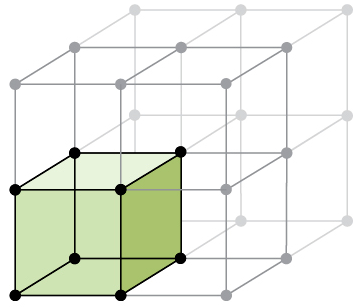Problem: The structure of manganese fluoride can be described as a simple cubic array of manganese ions with fluoride ions at the center of each edge of the cubic unit cell. What is the charge of the manganese ions in this compound?

FREE Expert Solution
87% (335 ratings)
Problem Details

The structure of manganese fluoride can be described as a simple cubic array of manganese ions with fluoride ions at the center of each edge of the cubic unit cell. What is the charge of the manganese ions in this compound?What scientific concept do you need to know in order to solve this problem?

Our tutors have indicated that to solve this problem you will need to apply the Unit Cell concept. You can view video lessons to learn Unit Cell Or if you need more Unit Cell practice, you can also practice Unit Cell practice problems .

What is the difficulty of this problem?

Our tutors rated the difficulty of The structure of manganese fluoride can be described as a si... as high difficulty.

How long does this problem take to solve?

Our expert Chemistry tutor, Dasha took 9 minutes to solve this problem. You can follow their steps in the video explanation above.

What professor is this problem relevant for?

Based on our data, we think this problem is relevant for Professor Arasasingham's class at UCI.

What textbook is this problem found in?

Our data indicates that this problem or a close variation was asked in . You can also practice practice problems .